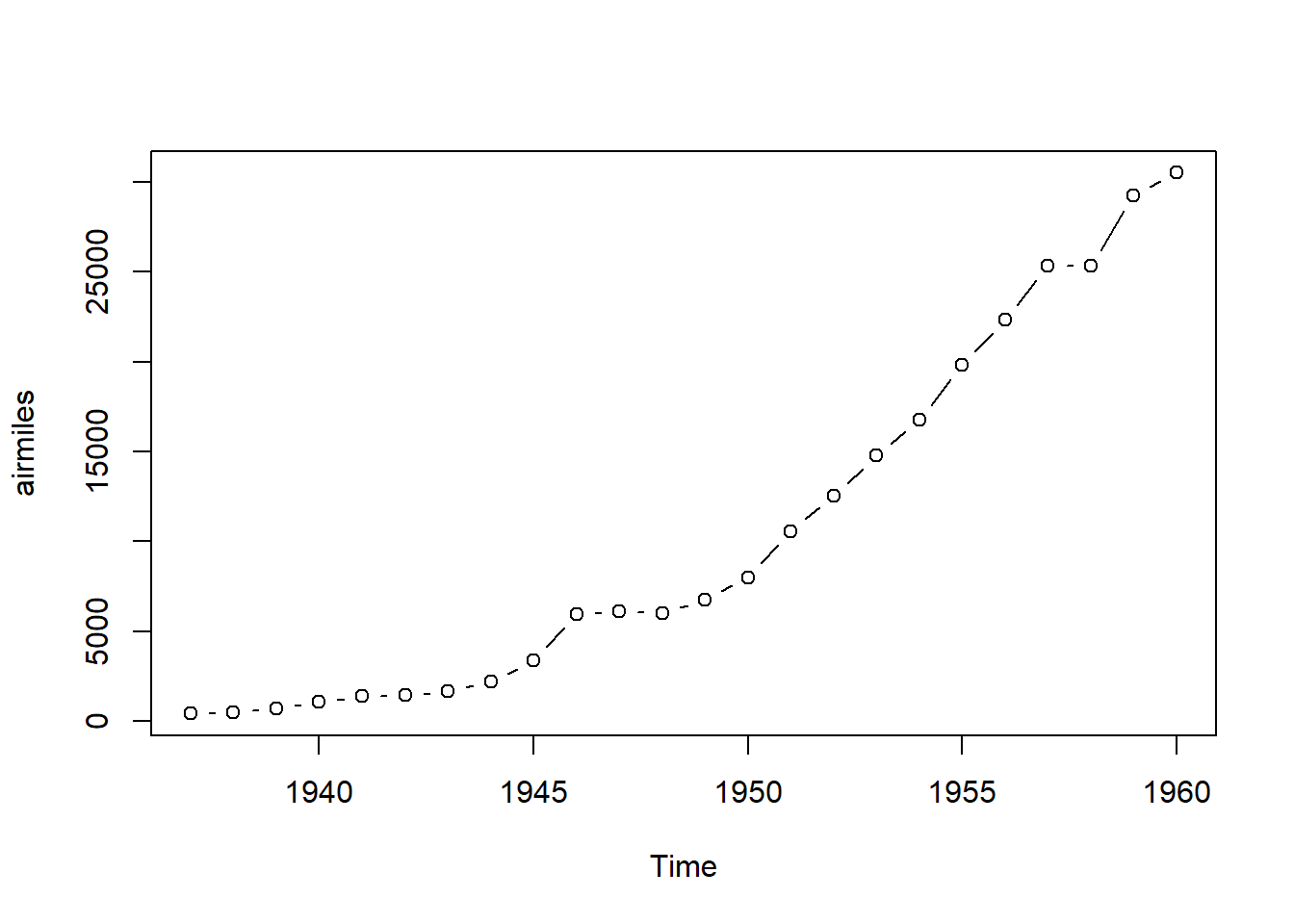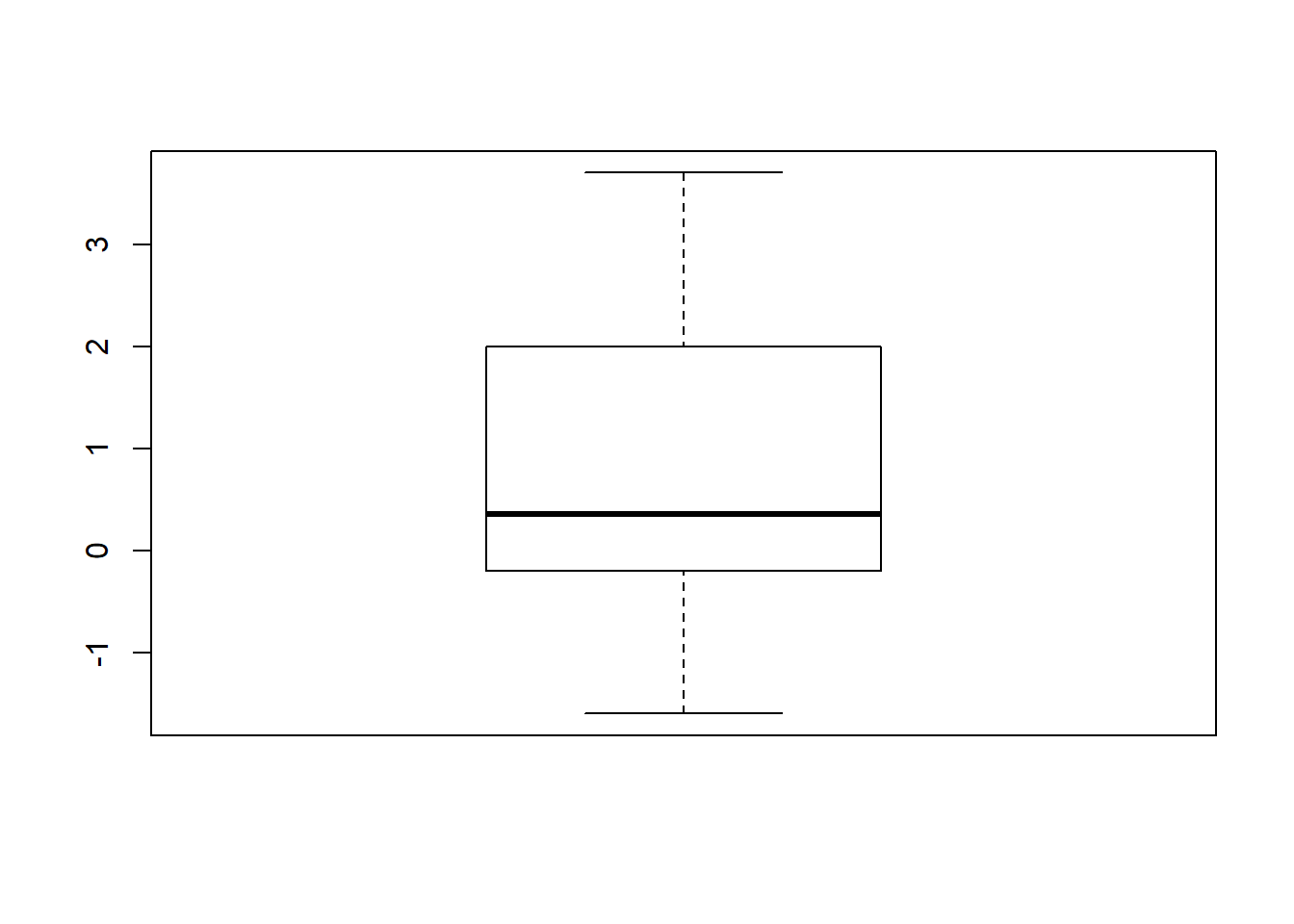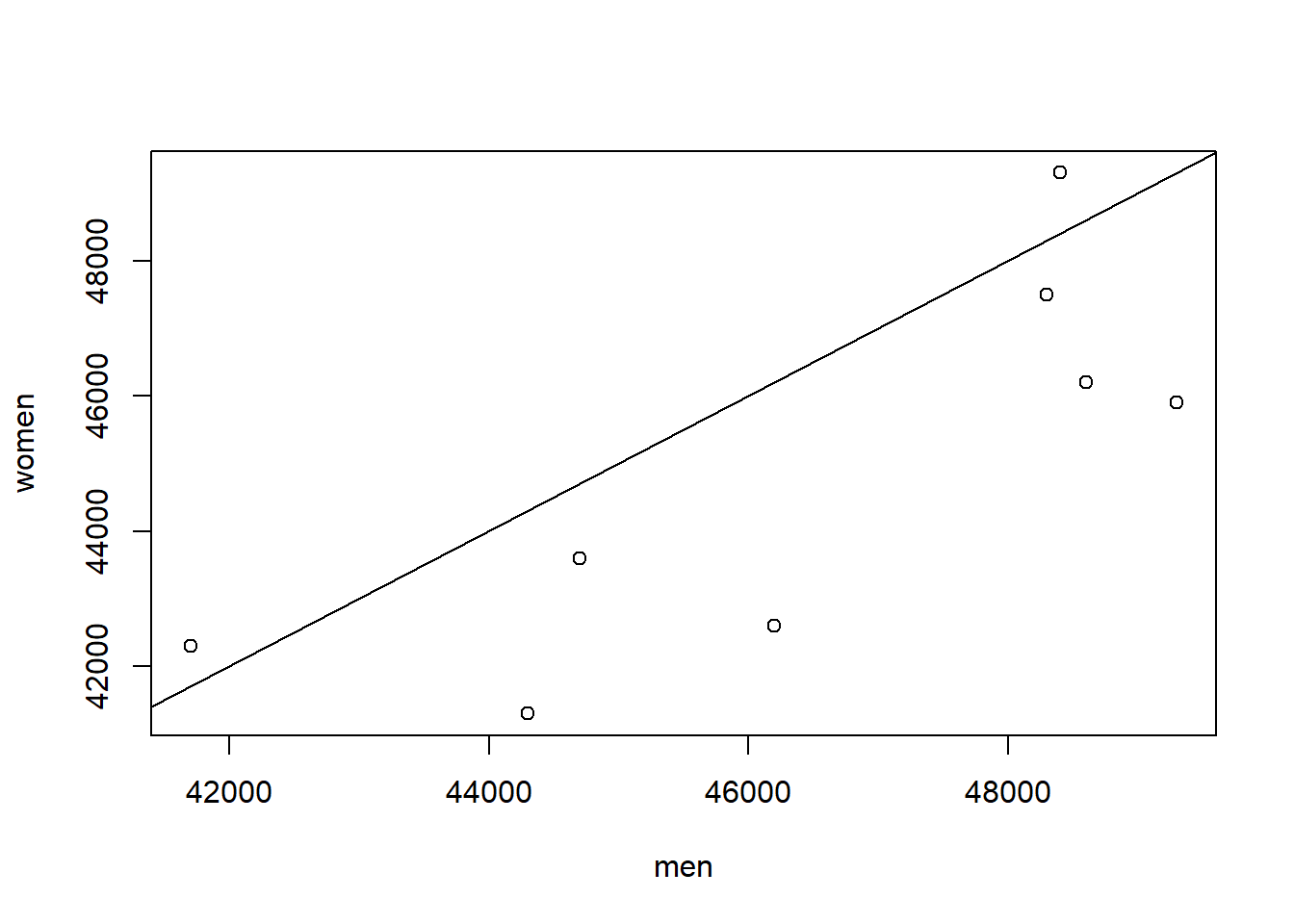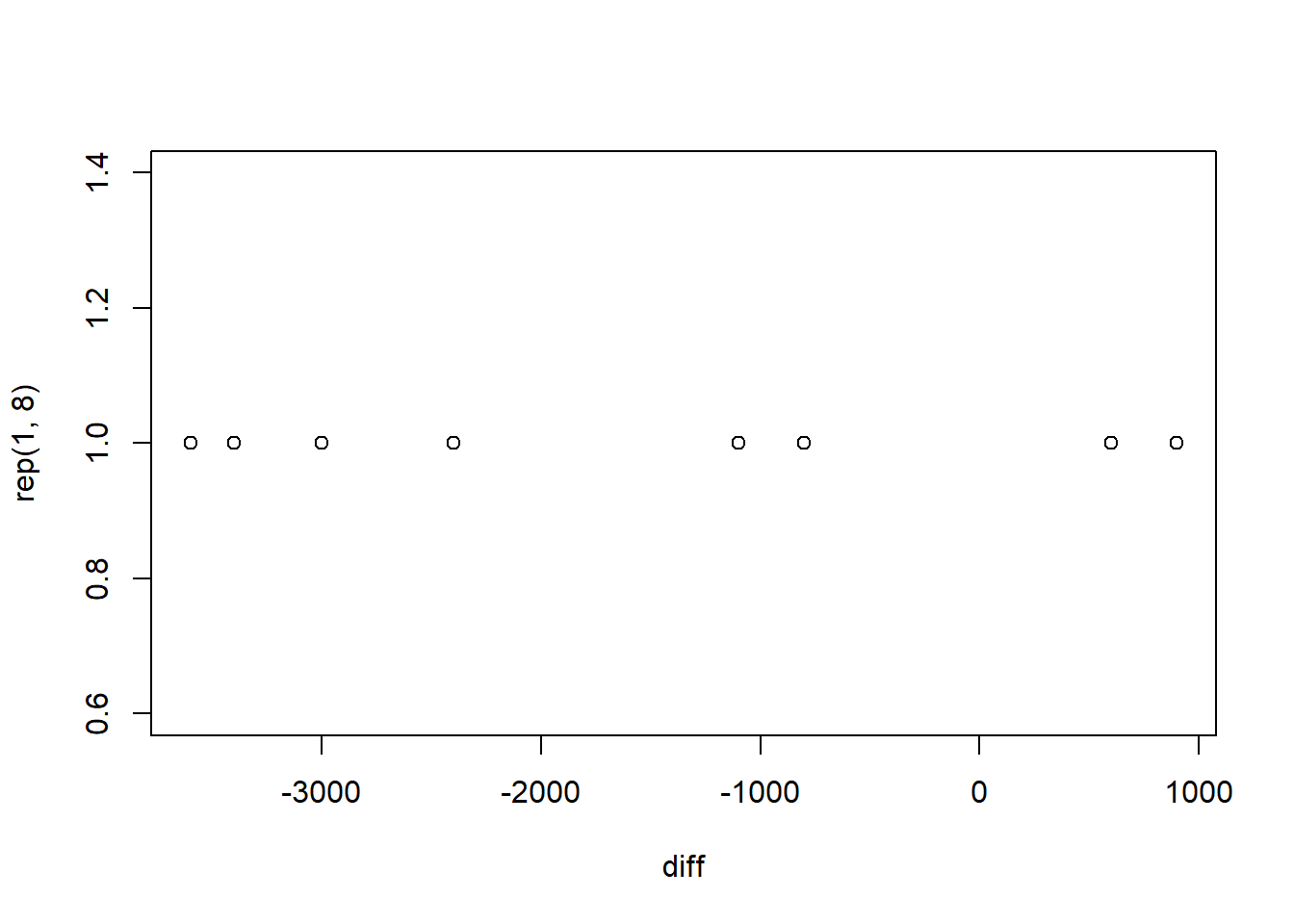### Homework exercise

To be solved at home before the exercise session.

1. Let $$x_1, \ldots , x_n$$ be a random sample (iid) from some distribution $$F_\theta$$ with the unknown parameter $$\theta$$. Which of the three one-sample tests ($$t$$-test, sign test or signed rank test) would you use (and why!) to test whether the location (expected value/median) of the data is equal to 1 if we know for certain that the distribution $$F_\theta$$ is
1. an exponential distribution with unknown rate parameter $$\theta$$,
2. a normal distribution with variance 2 and unknown expected value $$\theta$$,
3. a Laplace distirbution with known scale parameter 5 and unknown location parameter $$\theta$$,
4. a Poisson distirbution with unknown parameter $$\theta$$?
# In each case we want to use a test which makes the strictest assumptions (such that they are still satisfied). This gives us maximal power (lowest Type 2 error rate), as we "use more information" about the data. See the lecture examples of week 3.
# The assumptions the three tests make besides iid data are:
# t-test: normality                                       <- strictest
# signed rank test: symmetric continuous distribution     <- less strict
# sign test: continuous distribution                      <- even less strict

# a.
# Exponential distributions are not symmetric -> sign test.

# b.
# t-test

# c.
# Laplace distributions are not normal but they are symmetric -> signed rank test.

# d.
# Poisson distribution is neither continuous nor symmetric so, being strict, none of the tests apply. However, sign test is regularly applied to discrete data as well (e.g. using the conventions of slide 3.7).
b. The data set airmiles lists the passenger miles flown by commercial airlines in the United States for each year from 1937 to 1960. To inspect whether the yearly passenger miles equal 10000 on average, a researcher performed a sign test to test the null hypothesis $med_x = 10000$ on significance level 5% with the results shown below and concluded that there is no evidence against the null hypothesis. Do you agree with the researcher's conclusion?
airmiles
## Time Series:
## Start = 1937
## End = 1960
## Frequency = 1
##     412   480   683  1052  1385  1418  1634  2178  3362  5948  6109
##   5981  6753  8003 10566 12528 14760 16769 19819 22362 25340 25343
##  29269 30514
# Sign test
binom.test(sum(airmiles > 10000), length(airmiles))
##
##  Exact binomial test
##
## data:  sum(airmiles > 10000) and length(airmiles)
## number of successes = 10, number of trials = 24, p-value = 0.5413
## alternative hypothesis: true probability of success is not equal to 0.5
## 95 percent confidence interval:
##  0.2210969 0.6335694
## sample estimates:
## probability of success
##              0.4166667
# The sign test assumes that the observations x1, x2, ... ,xn form an iid. sample from some particular continuous distribution. While it could be plausible to view the yearly passenger miles as realizations of identically distributed random variables from a continuous distribution, they are certainly not independent. If the passenger miles go up one year, it is likely that they continue going up in the coming years as well (the technology develops etc.). This can be seen in the time series plot of the data:
plot(airmiles, type = "b")# In this kind of situation methods of time series analysis are needed (not covered in this course).

### Class exercise

To be solved at the exercise session.

Note: all the needed data sets are either given below or available in base R.

1. The data set sleep shows the effect of two soporific drugs (increase in hours of sleep compared to control) on 10 patients. We are interested in studying whether drug 1 helps in increasing the number of hours slept compared to placebo.
1. Extract the increases in hours of sleep of the patients who received drug 1 (group == 1).
2. Visualize the data.
3. Conduct an appropriate test to evaluate whether the location (expected value/median) of the increase in hours of sleep differs from 0 on significance level 5%.
4. Draw conclusions.
# a.
sleep_1 <- sleep[sleep$group == 1, 1] # b. boxplot(sleep_1)# c. # With so few observations it is difficult to say whether the data comes from a normal, or even a symmetric, distribution. Sign test seems like the safest choice. binom.test(sum(sleep_1 > 0), length(sleep_1)) ## ## Exact binomial test ## ## data: sum(sleep_1 > 0) and length(sleep_1) ## number of successes = 5, number of trials = 10, p-value = 1 ## alternative hypothesis: true probability of success is not equal to 0.5 ## 95 percent confidence interval: ## 0.187086 0.812914 ## sample estimates: ## probability of success ## 0.5 # p-value = 1 # The highest possible p-value -> no evidence against H0 -> the drug 1 is no better than placebo. 1. The data set below contains the annual salaries (in dollars) of 8 American women and 8 American men (recall exercise 3.2). The observations are paired such that each woman is matched with a man having similar background (age, occupation, level of education, etc). We are interested in studying whether the locations of the salaries of women and men differ (recall that last time paired $$t$$-test concluded that the salaries differ) . 1. Begin again by visualizing the data. 2. Which two non-parametric tests are appropriate in studying our question of interest? 3. State the hypotheses of the tests and conduct them on the significance level 10%. 4. What are the conclusions of the tests? 5. What assumptions did the test in part c make? Are they justifiable? salary <- data.frame(women = c(42600, 43600, 49300, 42300, 46200, 45900, 47500, 41300), men = c(46200, 44700, 48400, 41700, 48600, 49300, 48300, 44300)) # a. salary <- data.frame(women = c(42600, 43600, 49300, 42300, 46200, 45900, 47500, 41300), men = c(46200, 44700, 48400, 41700, 48600, 49300, 48300, 44300)) # Alternative visualization to the last time plot(women ~ men, data = salary) abline(a = 0, b = 1)# Most points are below the y=x -line, meaning that the salary of the man in a pair is more often larger than that of the woman # b. # The data is paired (and the pairs are not independent), making paired sign test and paired signed rank test appropriate choices. # Note that using a two-sample rank test is not justified as it assumes the independence of the two samples. # c. # Both tests have the same hypotheses # H0: med_(women - men) == 0 # H1: med_(women - men) != 0 diff <- salary$women - salary\$men

# Paired sign test
binom.test(sum(diff > 0), length(diff))
##
##  Exact binomial test
##
## data:  sum(diff > 0) and length(diff)
## number of successes = 2, number of trials = 8, p-value = 0.2891
## alternative hypothesis: true probability of success is not equal to 0.5
## 95 percent confidence interval:
##  0.03185403 0.65085579
## sample estimates:
## probability of success
##                   0.25
# Paired signed rank test
wilcox.test(diff, mu = 0)
##
##  Wilcoxon signed rank test
##
## data:  diff
## V = 4, p-value = 0.05469
## alternative hypothesis: true location is not equal to 0
# d.
# Paired sign test does not reject the null but the paired signed rank test does.

# e.
# Both tests assume that the differences d1, d2, ... ,d8 form an iid. sample from some particular continuous distribution. Paired signed rank test furthermore assumes that this distribution is symmetric. It is difficult to say whether the underlying distribution is symmetric based on a so small sample so the paired sign test might be a better choice -> no difference in salaries.
plot(diff, rep(1, 8))1. Eight female Aalto students and twelve male Aalto students were chosen randomly and lined up based on their heights (shortest first). The sexes (female/male) in the line have the pattern shown below. Use two-sample rank test to study whether the median height of females differs from the median height of males on significance level 5%.
1. Write down the assumptions and the hypotheses of the test.
2. Do you think the assumptions are plausible in this case?
3. Create two new vectors, female which contains the ranks of the females in the line and male which contains the ranks of the males in the line.
4. Conduct the two-sample rank test and draw conclusions.
line <- c("F", "F", "M", "M", "F", "M", "F", "F", "M", "F", "M", "F", "M", "M", "M", "F", "M", "M", "M", "M")
# a.
# The test assumes that the female and male samples are mutually independent iid samples from the continuous distributions Fx and Fy, respectively. Moreover, the distributions Fx and Fy are assumed to be equal up to location shift ("same-shaped hills").

# The null hypothesis is that the medians of Fx and Fy (and consequently the distributions itself) are equal (and the alternative hypothesis is the opposite of that).

# b.
# As the samples where chosen randomly, it is plausible that the samples are independent and iid. Also, googling "female vs. male height distribution" shows that the male distribution of heights is slightly wider than for females. We assume that this difference in scales is small enough that the test can still be used.

# c.
line <- c("F", "F", "M", "M", "F", "M", "F", "F", "M", "F", "M", "F", "M", "M", "M", "F", "M", "M", "M", "M")

female <- (1:20)[line == "F"]
male <- (1:20)[line == "M"]

# d.
wilcox.test(female, male)
##
##  Wilcoxon rank sum test
##
## data:  female and male
## W = 25, p-value = 0.0825
## alternative hypothesis: true location shift is not equal to 0
# p-value = 0.0825 -> not enough evidence to reject H0 on significance level 5% -> no difference in medians. As we "know" that there should be a difference, then either the sample size was too small, the result was caused by randomness or the assumptions weren't justified.

1. (Optional) Data manipulation using just functions in base R does not always produce the most readable code. The task in 1a. can be achieved more transparently using the package dplyr as follows.
# install.packages("dplyr")
library(dplyr)
sleep_1 <- sleep %>%
filter(group == 1) %>%
select(extra)

Find out how the package and the piping operator %>% work by going through an online tutorial.